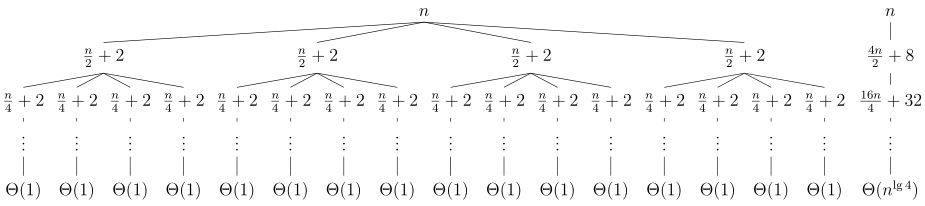## Exercise 4.4-3

Use a recursion tree to determine a good asymptotic upper bound on the recurrence $$T(n) = 4T(n/2 + 2) + n$$. Use the substitution method to verify your answer.

The recurrence $$T(n) = 4T(n/2 + 2) + n$$ has the following recursion tree:Adding up the costs of each level of the tree:

$\begin{split} T(n) & = n + \frac{4n}{2} + 8 + \frac{16n}{4} + 32 + \cdots + \Theta(n^{\lg4}) \\ & = \sum\limits_{i=0}^{\lg(n-1)} 2^i n + 2 \sum\limits_{i=0}^{\lg(n-1)} 4^i + \Theta(n^2) \\ & = \frac{2^{\lg n} - 1}{2 - 1}n + 2 \cdot \frac{4^{\lg n} - 1}{4 - 1} + \Theta(n^2) \\ & = (2^{\lg n} - 1)n + \frac{2}{3}(4^{\lg n} - 1) + \Theta(n^2) \\ & = (n - 1)n + \frac{2}{3}(n^2 - 1) + \Theta(n^2) \\ & = \Theta(n^2) \\ \end{split}$

Based on this calculation, we guess that $$T(n) \leq cn^2 - bn$$. Substituting this into the recurrence yields

$\begin{split} T(n) & \leq 4(c(n/2 + 2)^2 - b(n/2 + 2)) + n \\ & = 4\left(\frac{cn^2}{4} + \frac{4cn}{2} + 4c - \frac{bn}{2} -2b\right) + n \\ & = cn^2 + 8cn + 16c - 2bn - 8b + n \\ & = cn^2 -bn + 8cn - bn + 16c - 8b + n \\ & = cn^2 -bn -(b - 8c - 1)n - 8(b - 2c) \\ & \leq cn^2 - bn \\ \end{split}$

Where the last step holds for $$b - 8c -1 \geq 0$$.

The following LaTeX code was used to generate the above recursion tree: